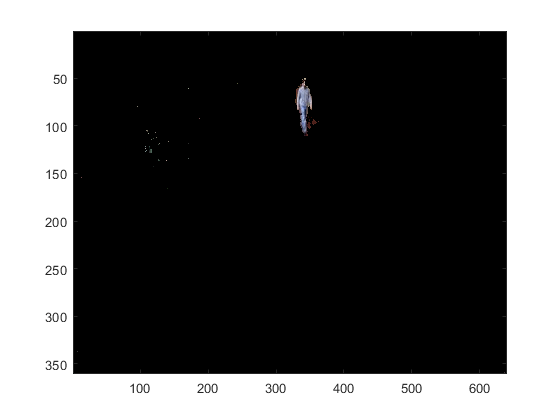# Subtract Image Background by Using OpenCV in MATLAB

This example shows how to subtract the background in an image sequence or a video by using the prebuilt MATLAB interface to the OpenCV function `cv::BackgroundSubtractorKNN`. In this example, you also use the `createMat` utility function to define the input and output arrays, and the `getImage` utility function to read the output image returned by the OpenCV function. The input video must be have a static background and dynamic foreground objects.

Read a video into the MATLAB workspace by using the `VideoReader` MATLAB function.

```videoSample = VideoReader("atrium.mp4"); videoSample.CurrentTime = 2.5;```

Add the MATLAB interface to OpenCV package names to the import list.

```import clib.opencv.*; import vision.opencv.*;```

Specify the parameter values to compute the background by using the OpenCV function for k-Nearest Neighbor (KNN) background subtractor `cv::BackgroundSubtractorKNN`. Set these values:

• Number of last frames to consider for computing the KNN background model `history` to `300`.

• Threshold for differentiating the foreground and background pixels `threshold` to `400`.

• `shadow` to `true`, to detect the shadow regions.

```history = 300; threshold = 400; shadow = true;```

Create a MATLAB interface object by using the `getBasePtr` utility function to represent the `BackgroundSubtractorKNN` class.

```cvPtr = cv.createBackgroundSubtractorKNN(history,threshold,shadow); kNNBase = util.getBasePtr(cvPtr);```

You can also set the parameter values for the background subtractor by accessing the public methods of the `BackgroundSubtractorKNN` class. Set the number of `k` nearest neighbors required for classifying a pixel as belonging to the background model to 2.

`kNNBase.setkNNSamples(2);`

Follow these steps to extract the foreground region by using the `apply` method of the OpenCV class `BackgroundSubtractorKNN`.

• Create an `InputArray` and `OutputArray` class by using the `createMat` MATLAB utility function to store the input video frame and the output foreground mask respectively.

• The `apply` method takes the video frames as inputs and then, computes the foreground pixels by using the k-NN algorithm. The `apply` method stores the mask containing the foreground pixel regions to the `OutputArray` class.

• Export the output foreground mask returned by the `apply` method to MATLAB workspace by using the `getImage` MATLAB utility function.

• Extract the desired foreground region by using the foreground mask and the input video frames.

```foregroundmask = zeros(videoSample.Height,videoSample.Width,videoSample.NumFrames); while hasFrame(videoSample) frame = readFrame(videoSample); [inMat,imgInput] = util.createMat(frame); [outMat,outImg] = util.createMat(); kNNBase.apply(imgInput,outImg); foregroundmask = util.getImage(outImg); foregroundmask = rescale(foregroundmask); foregroundmask = cast(foregroundmask,"like",frame); foreground(:,:,1) = frame(:,:,1).*foregroundmask; foreground(:,:,2) = frame(:,:,2).*foregroundmask; foreground(:,:,3) = frame(:,:,3).*foregroundmask; image(foreground,Parent=gca); pause(0.01); end```